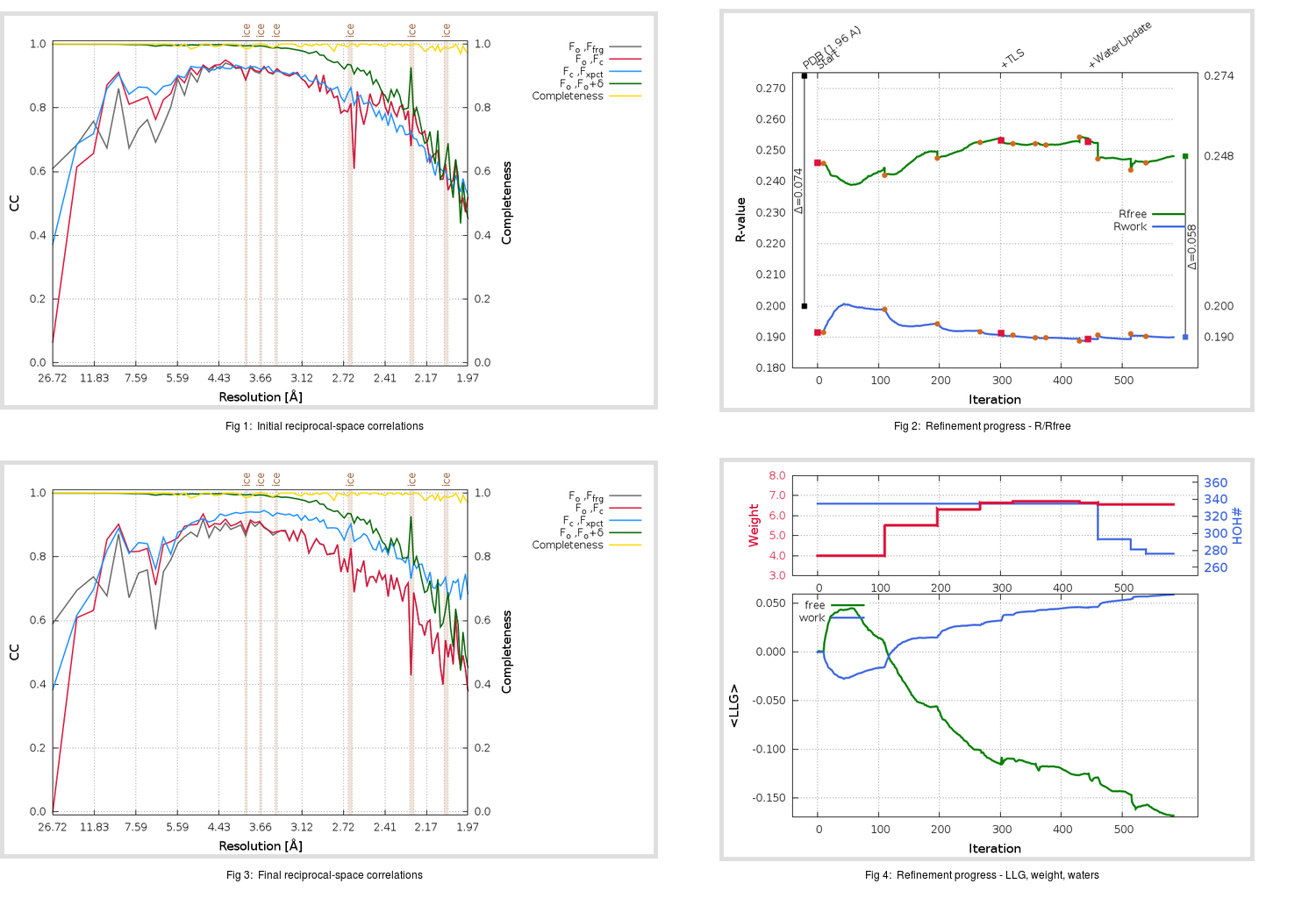Content:

```    Diffraction limits & principal axes of ellipsoid fitted to diffraction cut-off surface:
2.092         0.7547   0.0000   0.6561       0.972 a* + 0.236 c*
1.988         0.0000   1.0000   0.0000       b*
1.865        -0.6561   0.0000   0.7547      -0.884 a* + 0.468 c*
```

## Deposited

` `
 Date deposited Date data collection Resolution R, Rfree 20200315 20200304 1.96 0.1960 0.2740

Molprobity (CCP4 7.0 version) summary:

```Ramachandran outliers =   0.33 %
favored =  96.03 %
Rotamer outliers      =   1.14 %
C-beta deviations     =     0
Clashscore            =   4.43
RMS(bonds)            =   0.0129
RMS(angles)           =   1.74
MolProbity score      =   1.54
Resolution            =   1.96
R-work                =   0.1960
R-free                =   0.2740
```

```Number of waters      =   335

<B> (all atoms) =   34.04 ( sd =   10.48 ) for       2735 non-hydrogen atoms
<B>   (protein) =   33.39 ( sd =   10.22 ) for       2370 non-hydrogen atoms
<B>     (water) =   38.81 ( sd =   11.86 ) for        335 non-hydrogen atoms
<B>    (others) =   39.53 ( sd =   12.92 ) for         30 non-hydrogen atoms

B min/max       (all non-hydrogen atoms) =   15.83 /  115.33
B min/max   (protein non-hydrogen atoms) =   18.79 /  113.37
B min/max     (water non-hydrogen atoms) =   15.83 /  115.33
B min/max     (other non-hydrogen atoms) =   31.88 /   59.48
```

## BUSTER (re-)refinement

` `

Molprobity (CCP4 7.0 version) summary:

```Ramachandran outliers =   0.33 %
favored =  97.35 %
Rotamer outliers      =   0.76 %
C-beta deviations     =     0
Clashscore            =   2.11
RMS(bonds)            =   0.0117
RMS(angles)           =   1.56
MolProbity score      =   1.11
Resolution            =   1.96
R-work                =   0.1900
R-free                =   0.2482
```

```Number of waters      =   276

<B> (all atoms) =   32.94 ( sd =    8.30 ) for       2676 non-hydrogen atoms
<B>   (protein) =   32.26 ( sd =    7.56 ) for       2370 non-hydrogen atoms
<B>     (water) =   38.89 ( sd =   11.50 ) for        276 non-hydrogen atoms
<B>    (others) =   37.60 ( sd =   14.32 ) for         30 non-hydrogen atoms

B min/max       (all non-hydrogen atoms) =   10.54 /   70.77
B min/max   (protein non-hydrogen atoms) =   21.01 /   70.77
B min/max     (water non-hydrogen atoms) =   10.54 /   64.15
B min/max     (other non-hydrogen atoms) =   29.53 /   57.77
```

Refinement progression:Results:

` `
 File Remark 5REY_aB_refine.01_03_refine.pdb.gz exact refinement commands are in header 5REY_aB_refine.01_03_refine.mtz.gz including original deposited data and several re-refinement map coefficients 5REY_aB_refine.01_03_BUSTER_model.cif.gz including any non-standard compound restraints 5REY_aB_refine.01_03_BUSTER_refln.cif.gz